# DBMS_OUTPUT Package in Oracle

## DBMS_OUTPUT Package in Oracle with Examples

In this article, I am going to discuss DBMS_OUTPUT Package in Oracle with Examples. Please read our previous article where we discussed the basic concepts of Oracle Supplied Packages.

##### DBMS_OUTPUT Package in Oracle

We have learned about the overview of the DBMS_OUTPUT. Now let’s try to learn about the DBMS_OUTPUT. The DBMS_OUTPUT package contains many procedures.

• PUT appends text from the procedure to the current line of the line output buffer.
• NEW_LINE places an end-of-line marker in the output buffer. When we use PUT, we have to use NEW_LINE to show the messages.
• PUT_LINE combines the action of PUT and NEW_LINE (to trim leading spaces).
• GET_LINE retrieves the current line from the buffer into a procedure variable.
• GET_LINES retrieves an array of lines into a procedure-array variable.
• ENABLE/DISABLE enables and disables calls to DBMS_OUTPUT procedures.

Note: The minimum buffer size is 2000 and the maximum buffer size is unlimited. The default is 20,000. An integer parameter between 2000 and 1000000 in the ENABLE procedure.

The DBMS_OUTPUT is not that important for developers because they deal with messages using the development application features.

#### Example: Alter Windows, Console Windows.

Let’s take a few examples and see the benefits of the DBMS_OUTPUT.

###### Example 1:
```begin
for i in 1..30000
loop
end loop;
end;
```

From the example, we can see the code starts with begin. We have for loop with an ‘I’ variable between 1 and 30000. We have Dbms_output.put_line(lpad(I,7,0)). This prints the 7 digits numbers. If the number in the I variables doesn’t contain 7 digits, then the other digits will be replaced by 0. This happens because we are using lpad(I,7,0). Let’s try to execute this example.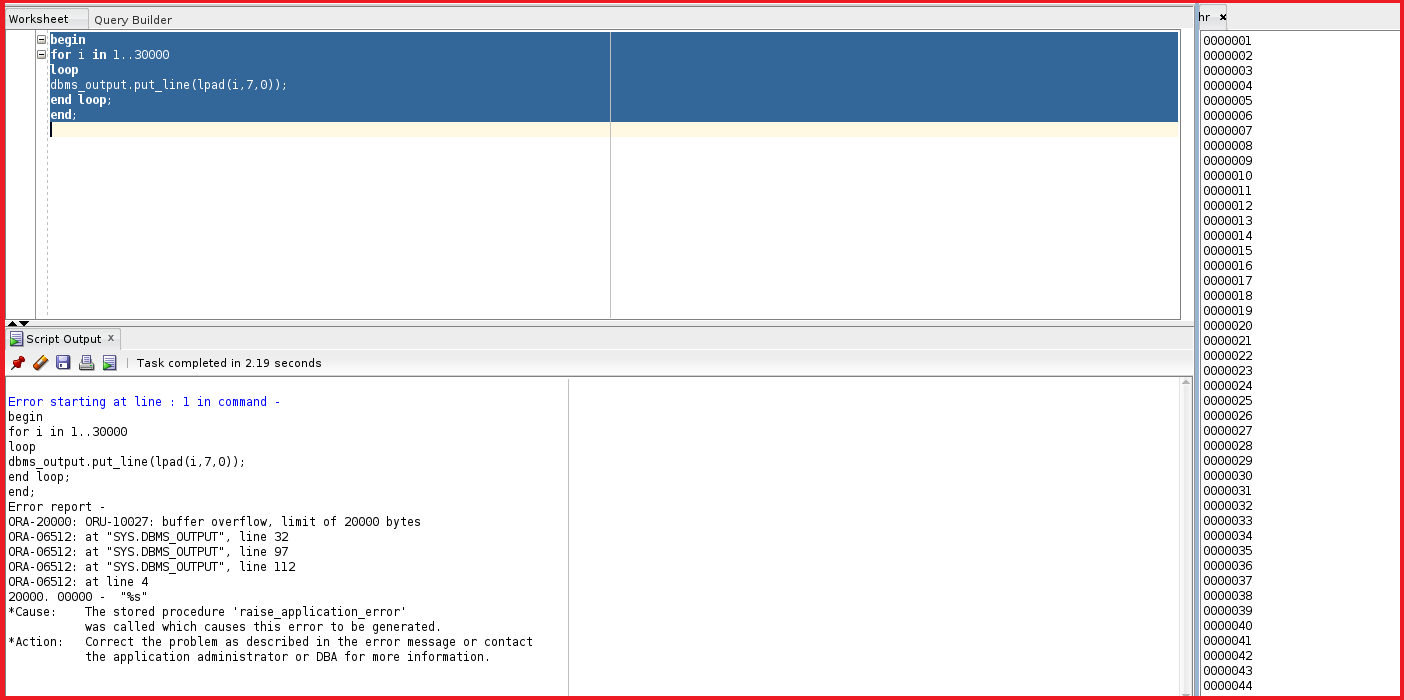We got an error showing buffer overflow. The limit of the buffer is 20000 bytes. In the DBMS_OUTPUT tab we can check the buffer size.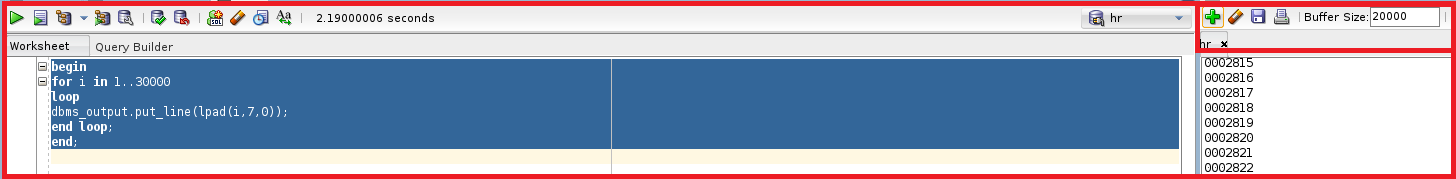The buffer size is 20000. The last number that is displayed is 0002857. Let’s have a look at the last displayed number.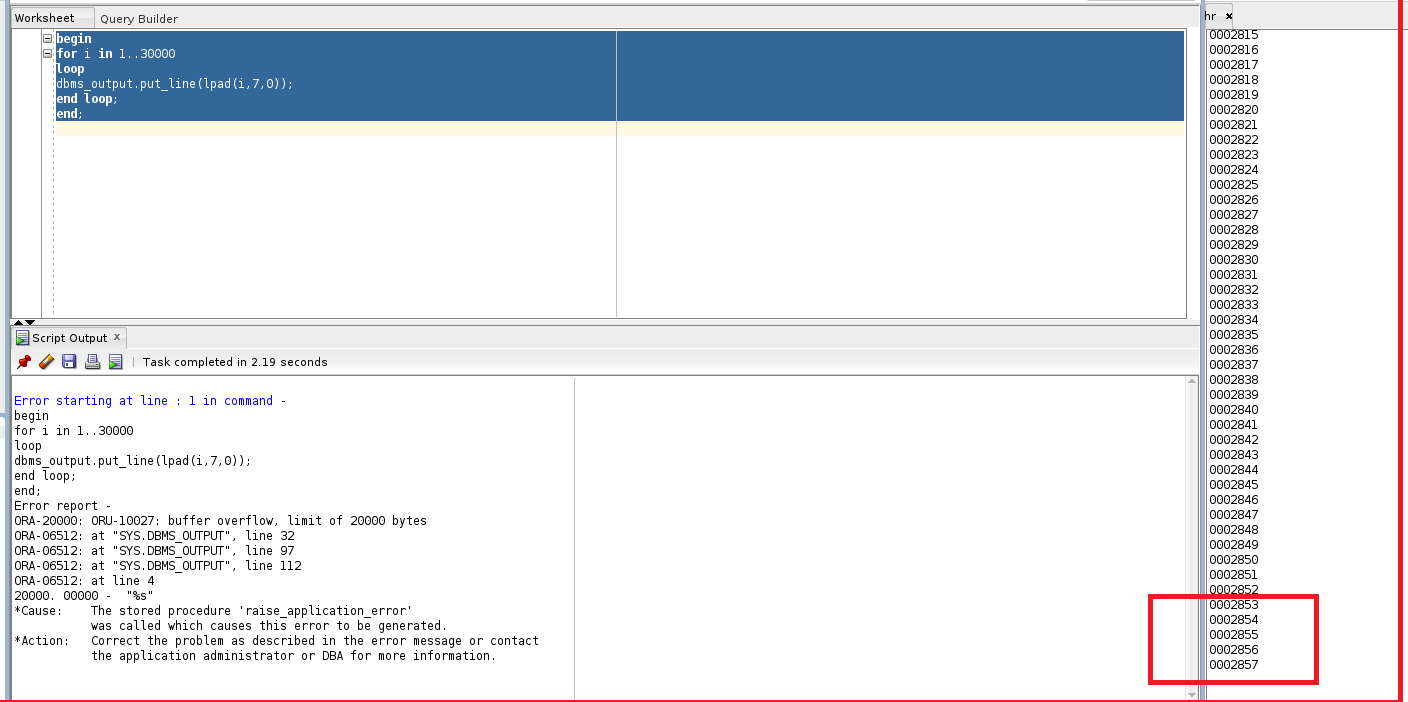The last displayed number is 0002857. If we multiply 0002587 by 9 you will be getting 19999. So, the dbms_output exceeded the buffer size. Let’s try to learn the second example which changes the dbms_output buffer size.

##### Example 2:
```begin
for i in 1..30000
loop
dbms_output.enable(1000000); --max 1,000,000
end loop;
end;
```

This is the same code but in the middle, this enables the buffer limit to 10,00,000. So, we are giving the maximum buffer size as 1 million. Now, let’s try to run this code.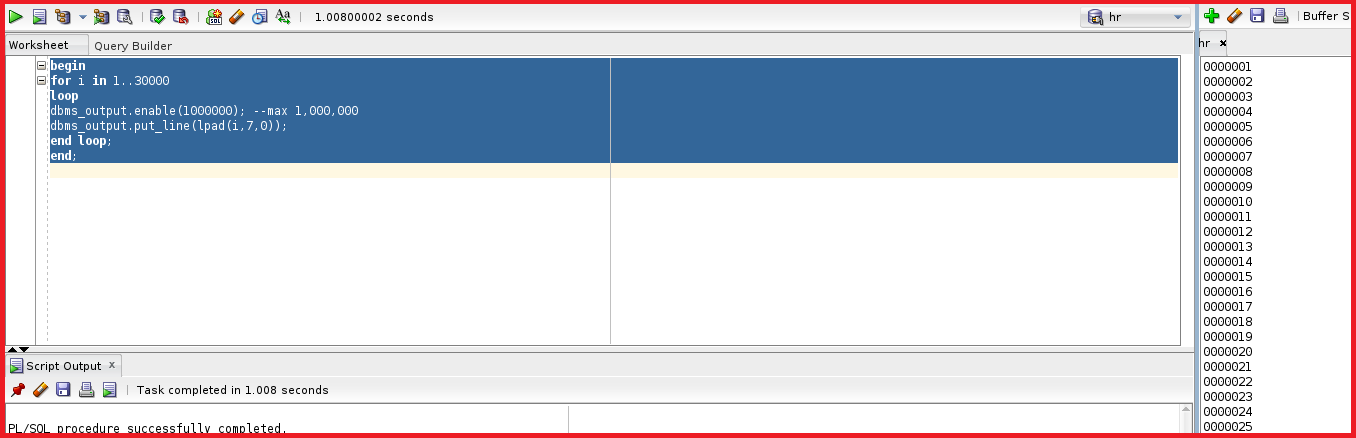So, the code is executed successfully. Let’s check the dbms_output whether we got the 30000 numbers or not.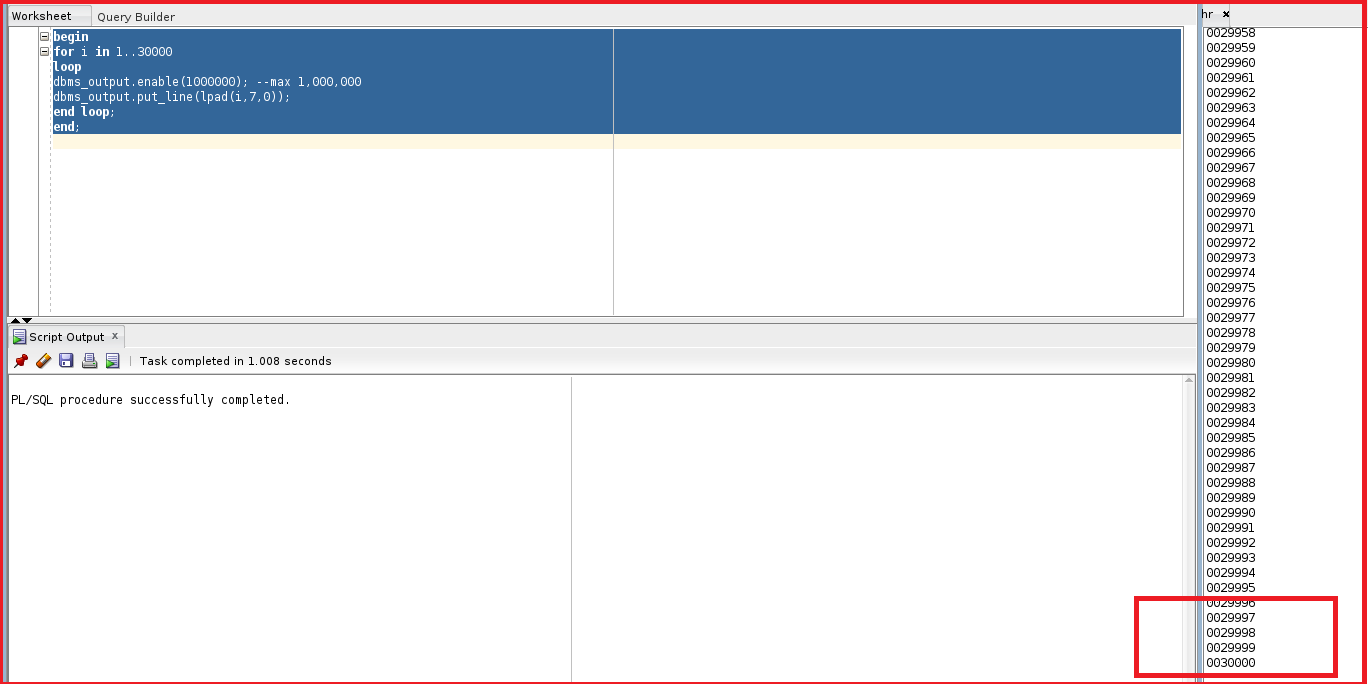So, this displayed all the 30000 numbers in the dbms_output. So, we can make changes to the buffer size. Let’s try to see the other example.

##### Example 3:
```begin
dbms_output.put_line('test');
dbms_output.disable;
dbms_output.put_line('test2');
dbms_output.enable;
dbms_output.put_line('test3');
end;
```

This is an example of disabling or enabling the buffer. This works with messages not sent until the PLSQL is completed. We have a procedure called disable and enable. We have used the package with procedure dbms_output.put_line(‘test’); After this, we have disabled the buffer so the ‘test’ will not be printed. After disabling we tried to print ‘test2’ and the test2 also doesn’t print because the buffer is disabled. Only test3 will be printed because it is executed after the buffer is enabled. Let’s try to run this code and see the output.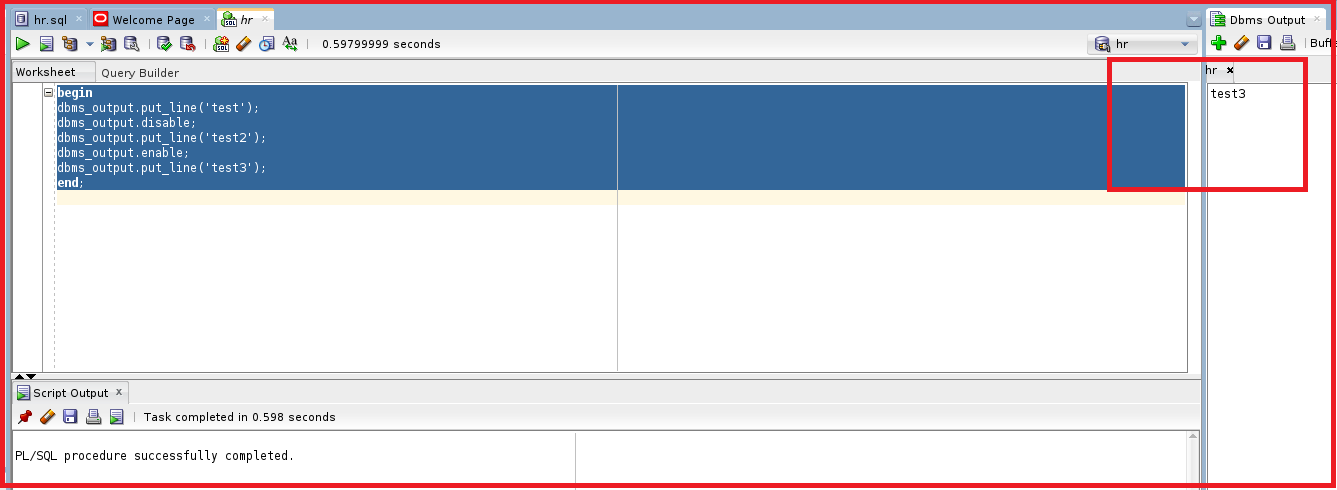From the output, we can see only the test 3 is printed. So, if the buffer is disabled then put_line does not work. If the buffer is enabled then the put_line will work. If the buffer is disabled it will discard and remove the old messages. Let’s try to look at the other example.

##### Example 4:
```begin
dbms_output.put_line('welcome');
dbms_output.put('my ');
dbms_output.put('name ');
dbms_output.put('is ');
dbms_output.put('jaya ');
dbms_output.new_line; --you should use this after put
dbms_output.put_line('my name is jaya');
end;
```

In this code, we have mentioned dbms_output.put(‘my’), name, is, jaya separately using the procedure put. We have used the new_line procedure in the dbms_output which is used to print all the above put sentences in one line. At last, we have used dbms_output.put_line(‘my name is jaya’); which is same as the above. Let’s try to execute this code.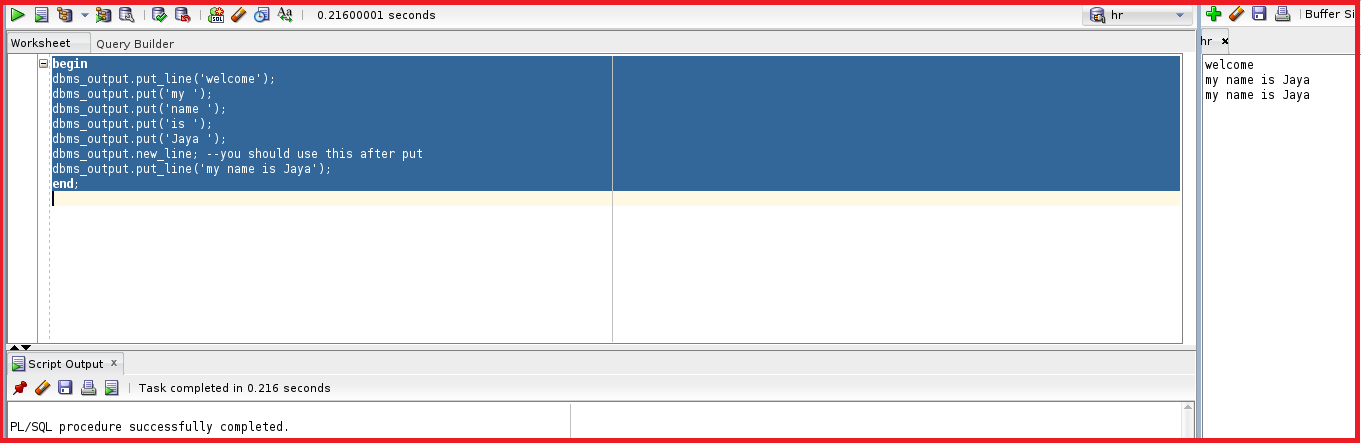We can see the output with the two lines is the same. So, all the put statements and the new_line statements are the same as the put_line statement. Put_line statements do include both put and line procedures.

So, this is how we play with DBMS_OUTPUT. We will understand about the GET_LINE procedure retrieves the single line buffer. In order to use the GET_LINE we have to define two variables with varchar2 and integer. Let’s have a look at the example.

##### Example 5:
```DECLARE
buffer VARCHAR2(100);
status INTEGER;
begin
dbms_output.put_line('line 1');
dbms_output.put_line('line 2');
dbms_output.put_line('line 3');
--This procedure retrieves a single line of buffered information.
dbms_output.get_line(buffer, status); --both are out parameters
dbms_output.put_line('Buffer: ' || buffer);
dbms_output.put_line('Status: ' || status);
/*If the call completes successfully,
then the status returns as 0. If there are no more
lines in the buffer, then the status is 1.
*/
end;
```

So, from the example, we have declared two variables buffer as varchar2 and status with integer datatypes. The code starts with begin. After beginning we are using three PUT_LINE procedures. These PUT_LINE will send the output to the buffer.

```dbms_output.put_line('line 1');
dbms_output.put_line('line 2');
dbms_output.put_line('line 3');
```

So, we are sending this to Buffer. After this, we will try to read this information in the buffer by using GET_LINE(‘Buffer:’,status). This means these two parameters are output parameters. The GET_LINE procedure retrieves a single line of the buffered information. Then we are sending the buffer parameter and status parameter to the buffer separately using PUT_LINE.

If the call completes successfully, then the status returns as 0. If there are no more buffers then the status is 1. Let’s execute this example.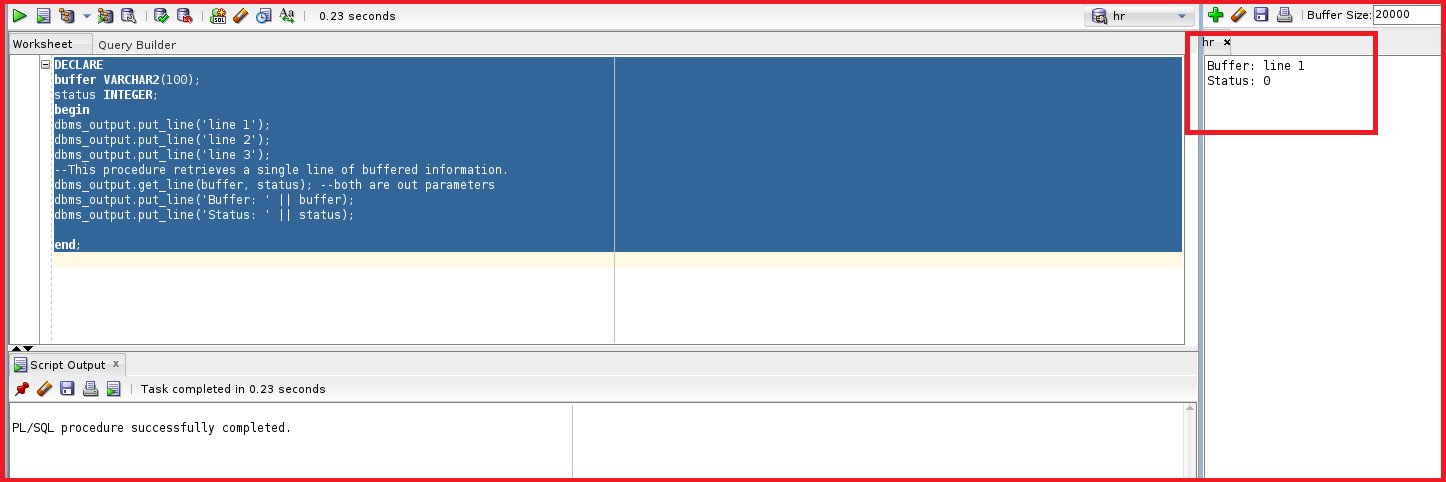The code is executed successfully. Even though we have mentioned the put_line procedure three times the output didn’t get reflected in the buffer. This is because we are using GET_LINE. When GET_LINE is used put_line will not be printed and GET_LINE takes the buffer from the PUT_LINE. The GET_LINE retrieves the single-line buffer information. So, the GET_LINE takes only the first line in the buffer. So, the buffer variable is stored with “Line 1”. So, even if we want both PUT_LINE and GET_LINE let’s look at another example.

##### Example 6:
```DECLARE
buffer VARCHAR2(100);
status INTEGER;
v VARCHAR2(3000);
begin
dbms_output.put_line('line 1');
dbms_output.put_line('line 2');
dbms_output.put_line('line 3');
for i in 1..3
loop
dbms_output.get_line(buffer, status);
v:=v||buffer||chr(10);
end loop;
dbms_output.put_line(v);
end;
```

So, from the above example. We have defined another variable v with varchar2(3000) as the datatype. The PUT_LINE procedure is the same as the previous one. After using the PUT_LINE procedure we are using the for loop for the GET_LINE procedure.

The GET_LINE in the for loop takes the first line in the buffer and the buffer will be concatenated with the variable v. In the same way, all three buffers will be concatenated with the variable v. Then in the final step we will try to print the variable v using dbms_output.put_line(v). Let’s try to execute the code.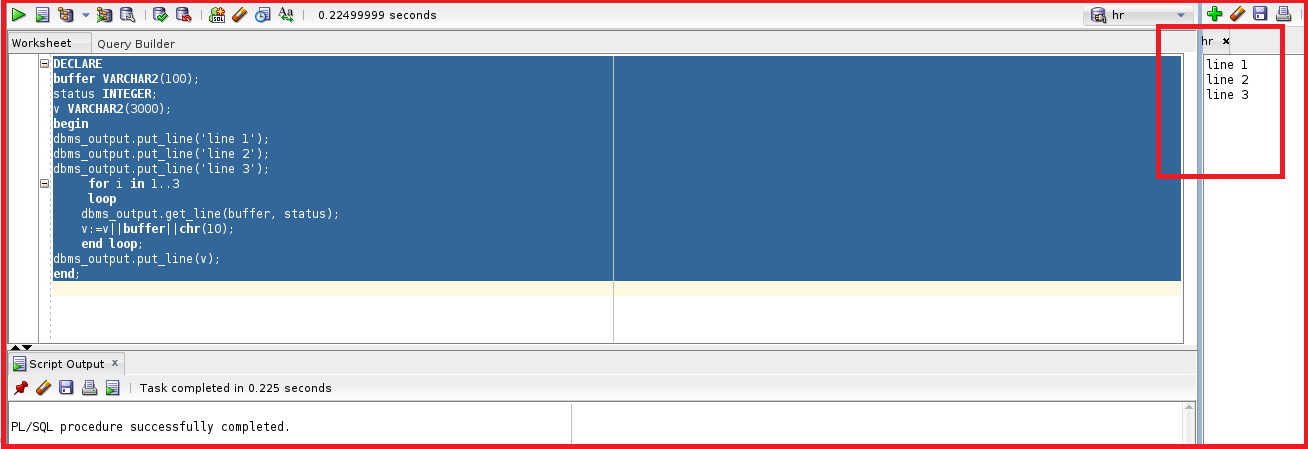So, we can see all three lines 1, line 2, and line 3 are printed successfully. Now, let’s have a look at the final example for the DBMS_OUTPUT.

##### Example 7:
```DECLARE
buffer DBMS_OUTPUT.CHARARR;
--  type chararr is table of varchar2(32767) index by binary_integer
v_line INTEGER;
begin

v_line:=10;
dbms_output.put_line('line 1');
dbms_output.put_line('line 2');
dbms_output.put_line('line 3');
dbms_output.put_line('line 4');
dbms_output.get_lines(buffer, v_line); --the first par is out, the second is in out
dbms_output.put_line( buffer(3) );
dbms_output.put_line( buffer(2) );
dbms_output.put_line( buffer(1 ));
dbms_output.put_line( buffer(4 ));

end;
```

The GET_LINES retrieves the array. The GET_LINES retrieves all the characters in the array. First, we can declare a variable buffer and the data type of the variable buffer is dbms_output.chararr which is the character array, and v_line with datatype as an integer. The variable buffer is a global variable with a type character array of varchar2(32767) and index by binary_integer. This indicates the variable buffer is an array.

In order to use GET_LINES we must declare two variables. The first variable is buffer which is array and the second variable is v_line which indicates how many we want to read the buffer.

We have mentioned v_line:=10 which indicates the GET_LINES procedure will read 10 lines. We have included dbms_output.put_line for line 1, line 2, line 3 and line 4.

After the put_line we have given get_lines(buffer, v_line). The first parameter is out and the second parameter is in-out. Here the GET_LINES indicates the oracle to put all the PUT_LINE into an array. So, after the array is filled, we can print the output index line.

After the GET_LINES we have mentioned the put_line with buffer(3), buffer(2), buffer(1) and finally buffer(4). Let’s try to execute the example.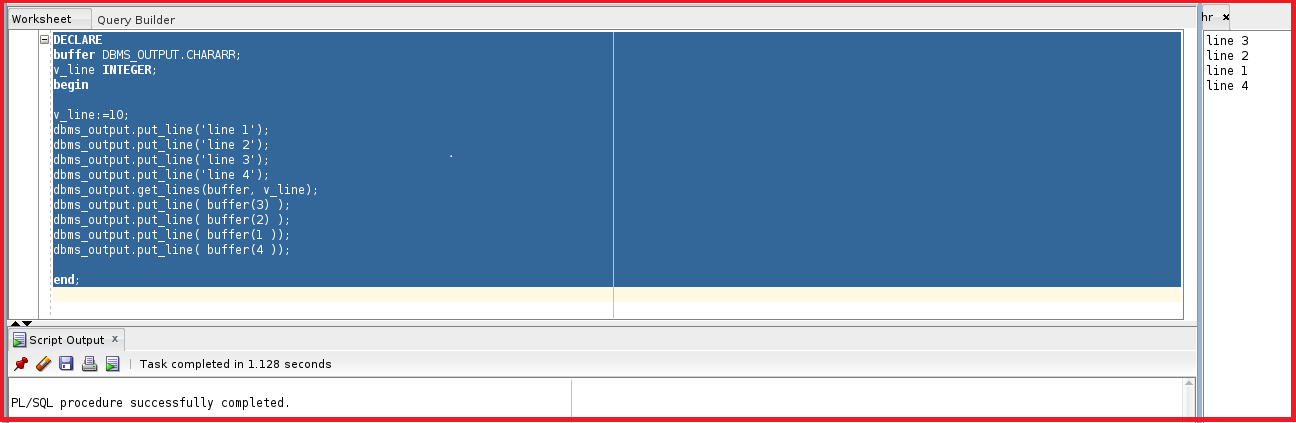The output is the same as we declared with the buffer. All this information is additional information for the best practice of Oracle PL/SQL. This is one of the Oracle Supplied Packages that we use in our daily life.

In the next article, I am going to discuss UTL_FILE Package in Oracle with Examples. Here, in this article, I try to explain DBMS_OUTPUT Package in Oracle with Examples. I hope you enjoy this DBMS_OUTPUT Package in the Oracle article.